## ML Aggarwal Class 8 Solutions for ICSE Maths Chapter 8 Simple and Compound Interest Ex 8.3

Question 1.
Calculate the amount and compound interest on
(i) ₹15000 for 2 years at 10% per annum compounded annually.
(ii) ₹156250 for $$1 \frac{1}{2}$$ years at 8% per annum compounded half yearly.
(iii) ₹ 100000 for 9 months at 4% per annum compounded quarterly.
Solution:
(i) Principal (P) = ₹15000
Rate (R) = 10% p.a.
Period (n) = 2 years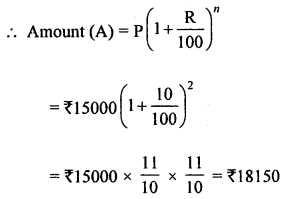∴ C.I. = A – P = ₹18150 – 15000 = ₹3150

(ii) Principal (P) = ₹156250
Rate (R) = 8% p.a. or 4% half-yearly
Period (n) = $$1 \frac{1}{2}$$ years = 3 half-year∴ C.I. = A – P = ₹175760 – ₹156250 = ₹19510

Question 2.
Find the difference between the simple interest and compound interest on ₹4800 for 2 years at 5% per annum, compound interest being reckoned annually.
Solution:
Principal (P) = ₹4800
Rate (R) = 5% p.a.
Period (n) = 2 yearsQuestion 3.
Find the compound interest on ₹3125 for 3 years if the rates of interest for the first, second and third year are respectively 4%, 5% and 6% per annum.
Solution:
Principal (P) = ₹3125
Rate of interest for continuous 3 years = 4%, 5%, 6%
Period (n) = 3 years∴ C.I. = A – P = ₹3617·25 – ₹3125 = ₹492·25

Question 4.
Kamla borrowed ₹26400 from a Bank to buy a scooter at a rate of 15% p.a. compounded yearly. What amount will she pay at the end of 2 years and 4 months to clear the loan?
Solution:
Sum borrowed (P) = ₹26400
Rate (R) = 15% p.a.
Period (n) = 2 years 2 monthsQuestion 5.
Anil borrowed ₹18000 from Rakesh at 8% per annum simple interest for 2 years. If Anil had borrowed this sum at 8% per annum compound interest, what extra amount would he have to pay?
Solution:
Sum borrowed (P) = ₹18000
Rate (R) = 8% p.a.
Time (n) = 2 years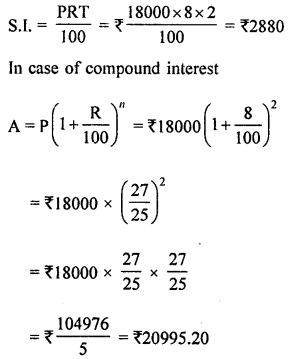∴ C.I. = ₹20995.20 – 18000 = ₹2995.20
Now difference in both interest = ₹2995.20 – ₹2880 = ₹115.20

Question 6.
Mukesh borrowed 775000 from a bank. If the rate of interest is 12% per annum, find the amount he would be paying after $$1 \frac{1}{2}$$ years if the interest is
(i) compounded annually
(ii) compounded half-yearly.
Solution:
Sum borrowed (P) = ₹75000
Rate (R) = 12% p.a. or 6% half-yearly
Period (n) = $$1 \frac{1}{2}$$ years or 3 half-years
(i) When the interest compounded yearly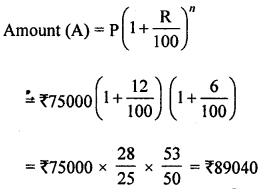(ii) When the interest compounded half-yearly, then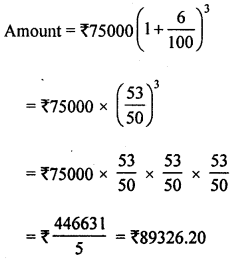Question 7.
Aryaman invested ₹10000 in a company, he would be paid interest at 7% per annum compounded annually. Find
(i) the amount received by him at the end of 2 years.
(ii) the interest for the 3rd year.
Solution:
Investment to a company (P) = ₹10000
Rate of interest (R) = 7% p.a.
Period (n) = 2 years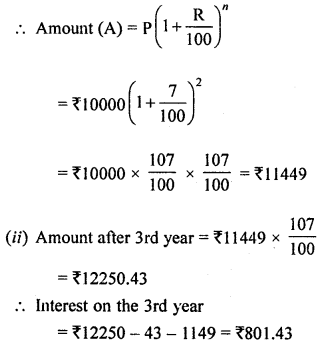Question 8.
What sum of money will amount to ₹9261 in 3 years at 5% per annum compound interest?
Solution:
Amount (A) = ₹9261
r = 5%, Time (t) = 3
P = ?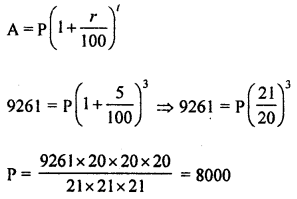Hence the sum of money = ₹8000

Question 9.
What sum invested for $$1 \frac{1}{2}$$ years compounded half-yearly at the rate 8% p.a. will amount to ₹ 140608?
Solution:
Amount (A) = ₹140608
Rate (R) = 8% p.a. = 4% half-yearly
Period (n) = $$1 \frac{1}{2}$$ years = 3 half-year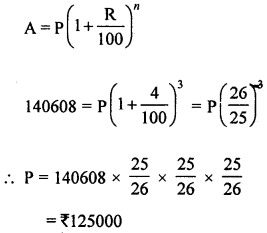∴ Principal = ₹ 125000

Question 10.
At what rate percent will ₹2000 amount to ₹2315·25 in 3 years at compound interest?
Solution:
Principal (P) = ₹2000
Amount (A) = ₹2315·25
Period (n) = 3 years
Let rate of interest = r % p.a.∴ r = $$\frac{100}{20}$$ = 5
∴ Rate of interest = 5% p.a.

Question 11.
If ₹40000 amounts to ₹46305 in $$1 \frac{1}{2}$$ years, compound interest payable half yearly, find the rate of interest per annum.
Solution:
Amount (A) = ₹17576
Principal (P) = ₹15625
Rate (R) = 4% p.a.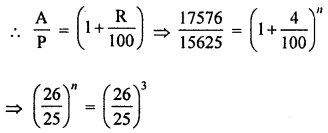Comparing, we get n = 3
∴ Time 3 years

Question 12.
In what time will ₹15625 amount to ₹ 17576 at 4% per annum compound interest?
Solution:
Amount (A) = ₹ 17576
Principal (P) = ₹15625
Rate = 4% p.a.
Let Period = n years
We know that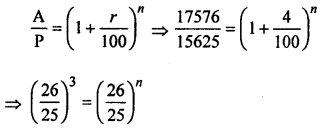∴ n = 3
Hence time = 3 years

Question 13.
₹ 16000 invested at 10% p.a. compounded semi-annually, amounts to ₹18522. Find the time period of investment.
Solution:
We know thatPrincipal (P) = ₹16000
Amount (A) = ₹18522
Rate = 10% p.a. or 5% semi-annually
Let period = n half-years
We know thatHence time = $$\frac{3}{2}=1 \frac{1}{2}$$ years